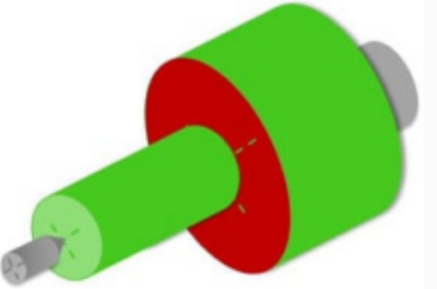## Download the free printable cheat sheet

have the important information always ready at your fingertips

Checkbox*Play Video

## GD&T Symbols

List of the different gd&t symbols and their meaning

Form tolerances

 Straightness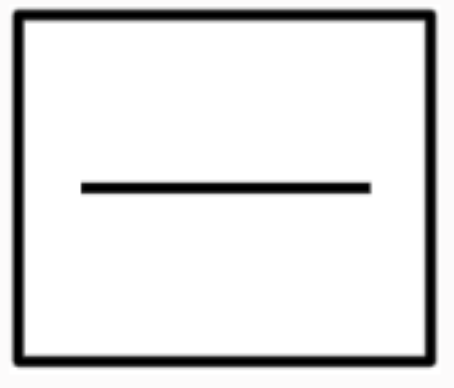The line that is pointed at by the arrow (leader) should lie between two Ideal lines that are parallel to each other, and the distance between these two planes is the value of the tolerance.Example of a drawing entry: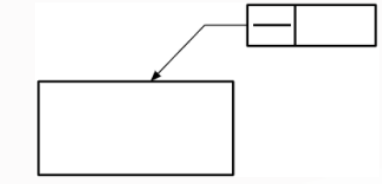The tolerancezone of this example would look like this: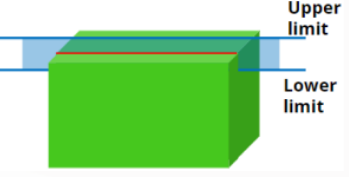red: the tolerated elementblue: the limits of the tolerance zone

 Flatness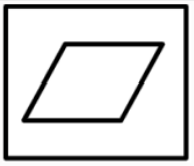The surface that is pointed at by the arrow (leader) should lie between two Ideal planes that are parallel to each other, and the distance between these two planes is the value of the tolerance.Example of a drawing entry: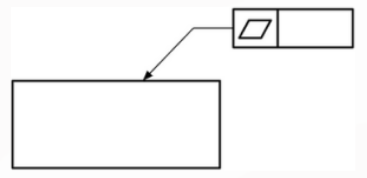The tolerancezone of this example would look like this: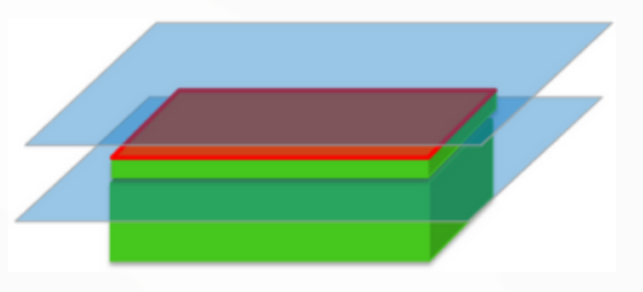red: the tolerated elementblue: the limits of the tolerance zone

 Circularity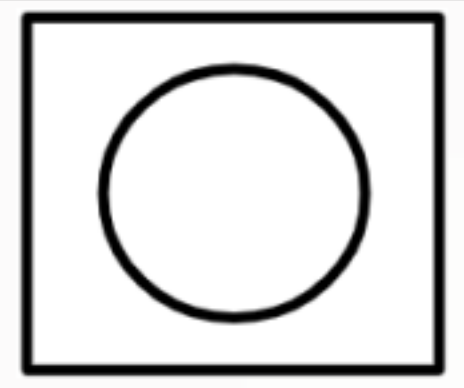The circle that is pointed at by the arrow (leader) should lie between two Ideal circles that are concentric with each other, and the distance between these two circles is the value of the tolerance.Example of a drawing entry: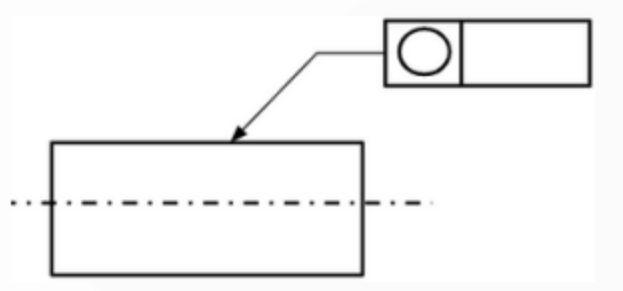The tolerancezone of this example would look like this: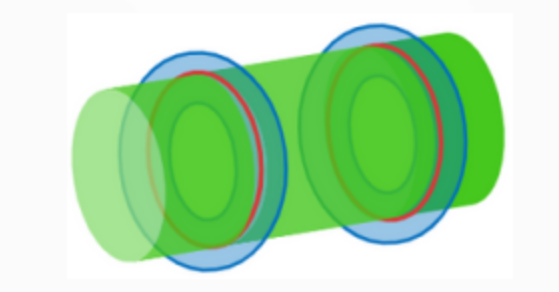red: the tolerated elementblue: the limits of the tolerance zone

 Cylindricity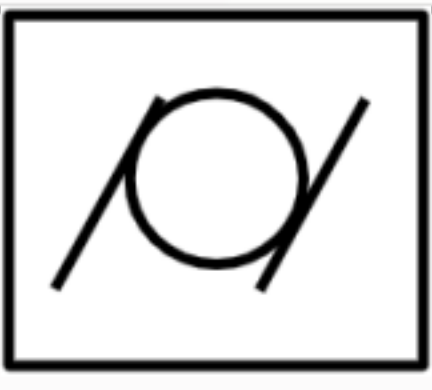The cylinder that is pointed at by the arrow (leader) should lie between two Ideal cylinders that are concentric with each other, and the distance between these two cylinders is the value of the tolerance.Example of a drawing entry: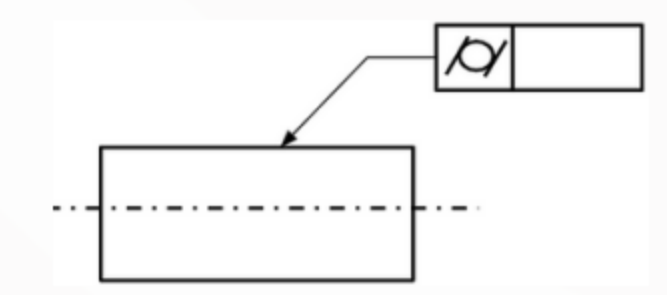The tolerancezone of this example would look like this: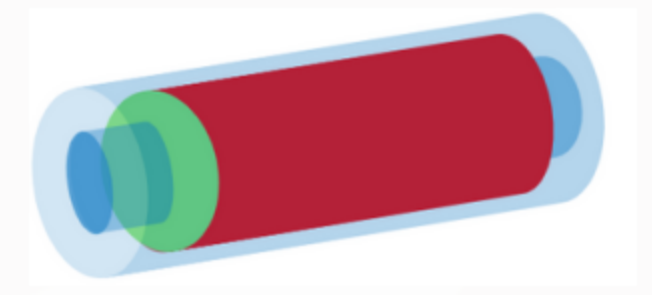red: the tolerated elementblue: the limits of the tolerance zone

Orientation tolerances

 Parallelism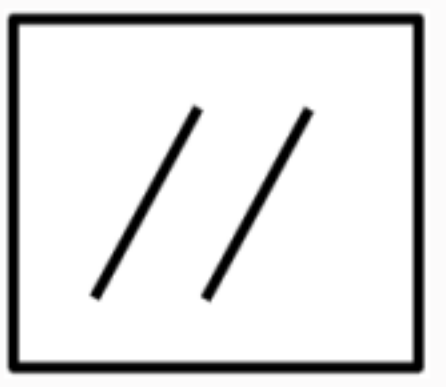The feature (line, centerline or planar surface) that is pointed at by the arrow (leader) should lie between two Ideal planes that are parallel to each other, and are ideally parallel to the datum and the distance between these two planes is the value of the tolerance.If there is a diameter sign added before the value of the tolerance, then the tolerance zone in this case is a cylinder, having a diameter equal to the tolerance value. the centerline of this cylinder is ideally parallel to the datum.Example of a drawing entry: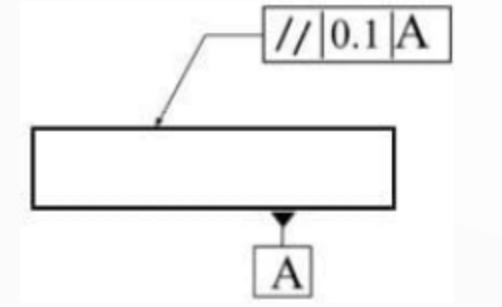The tolerance zone of this example would look like this: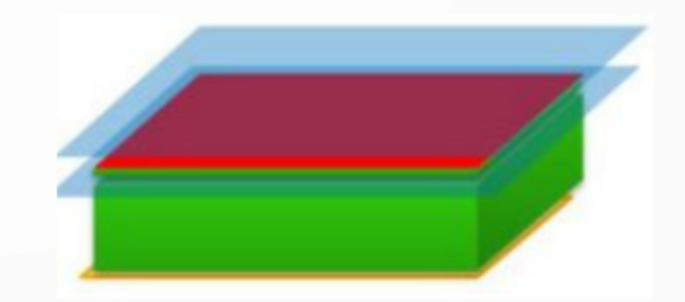red: the tolerated elementblue: the limits of the tolerance zoneyellow: the datum (the reference)

 Perpendicularity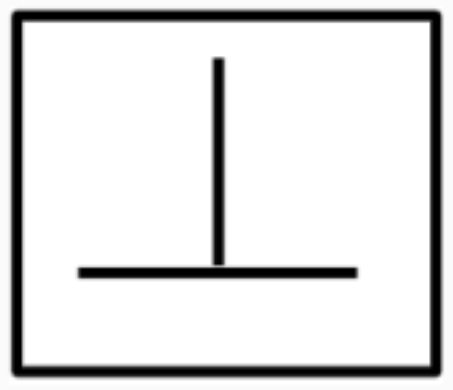The feature (line, centerline or planar surface) that is pointed at by the arrow (leader) should lie between two Ideal planes that are parallel to each other, and are ideally perpendicular to the datum and the distance between these two planes is the value of the tolerance.If there is a diameter sign added before the value of the tolerance, then the tolerance zone in this case is a cylinder, having a diameter equal to the tolerance value. the centerline of this cylinder is ideally perpendicular to the datum.Example of a drawing entry: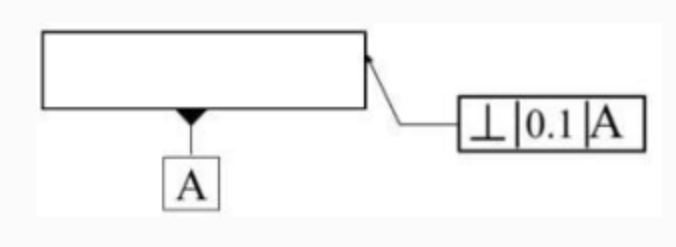The tolerancezone of this example would look like this: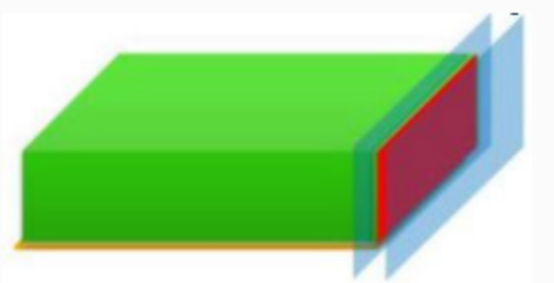red: the tolerated elementblue: the limits of the tolerance zoneyellow: the datum (the reference)

 Angularity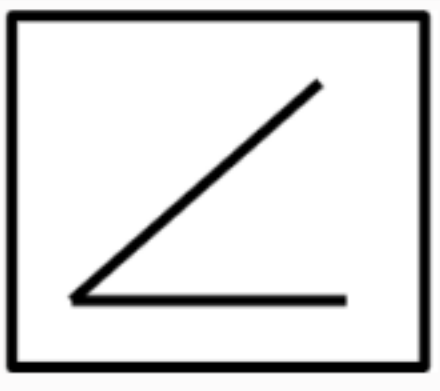The feature (line, centerline or planar surface) that is pointed at by the arrow (leader) should lie between two Ideal planes that are parallel to each other, and have a theoritically exact angle to the datum and the distance between these two planes is the value of the tolerance.If there is a diameter sign added before the value of the tolerance, then the tolerance zone in this case is a cylinder, having a diameter equal to the tolerance value. the centerline of this cylinder has a theoreticaly exact angle to the datum.Example of a drawing entry: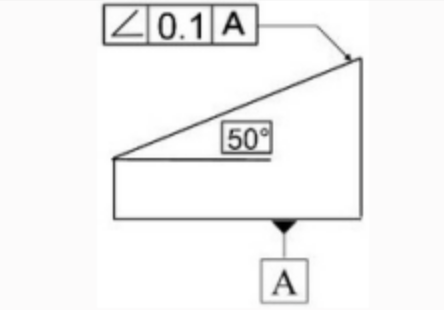The tolerancezone of this example would look like this: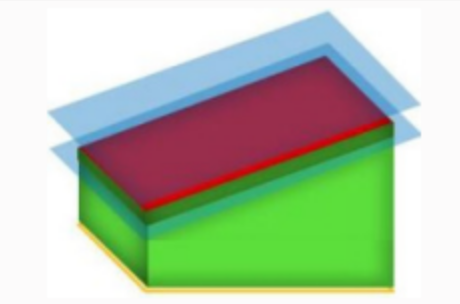red: the tolerated elementblue: the limits of the tolerance zoneyellow: the datum (the reference)

 Profile of a line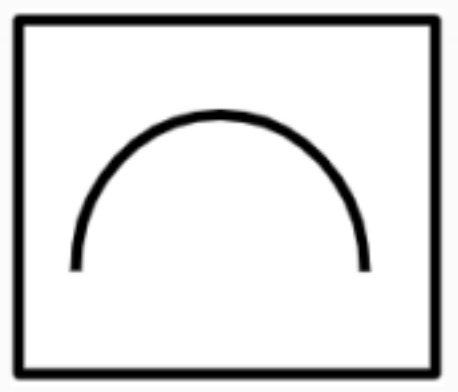Any line on the profile that is pointed at by the arrow (leader) should lie between two Ideal linear profiles that are offset from each other, and the distance between these two profiles is the value of the tolerance.Example of a drawing entry: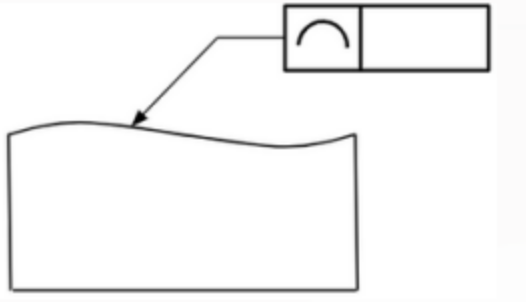The tolerancezone of this example would look like this: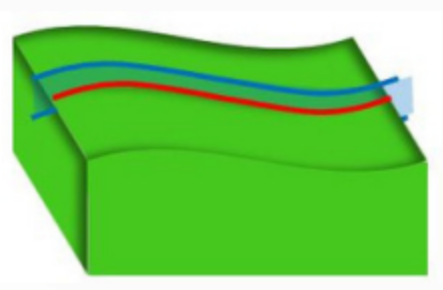red: the tolerated elementblue: the limits of the tolerance zone

 Profile of a surface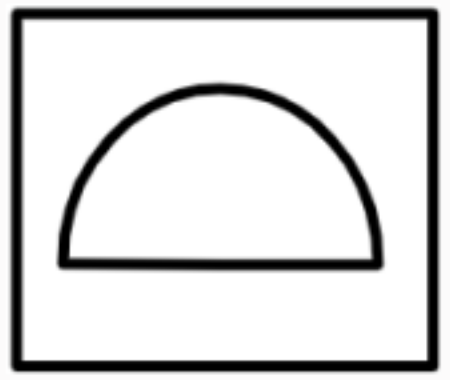The profile that is pointed at by the arrow (leader) should lie between two Ideal profiles that are offset from each other, and the distance between these two profiles is the value of the tolerance.Example of a drawing entry: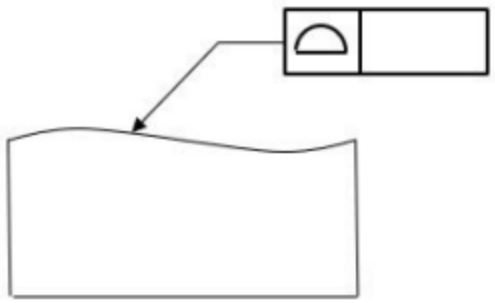The tolerancezone of this example would look like this: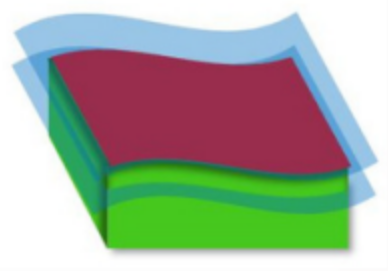red: the tolerated elementblue: the limits of the tolerance zone

Location tolerances

 Position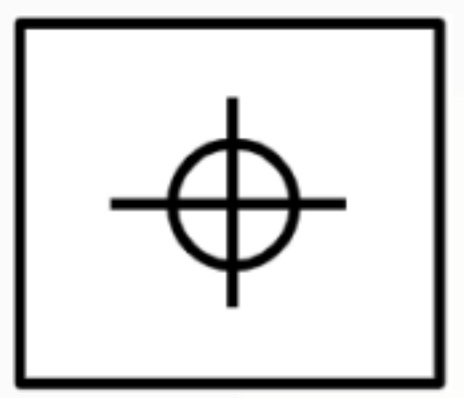Position specifies that the tolerated feature should lie in a tolerance zone which has a certain location in the part with respect to other features. Position needs a datum and theoretical dimensions. So this specification means that the tolerated centerline of the hole should lie inside a tolerance zone which is a circle with diameter of 0.1. The position of the centerline of this circle lies in a distance of 10 to datum A and 10 to datum B Example of a drawing entry: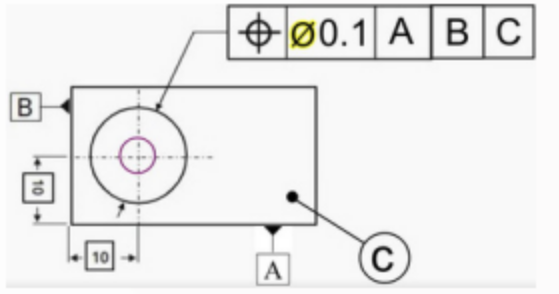The tolerancezone of this example would look like this: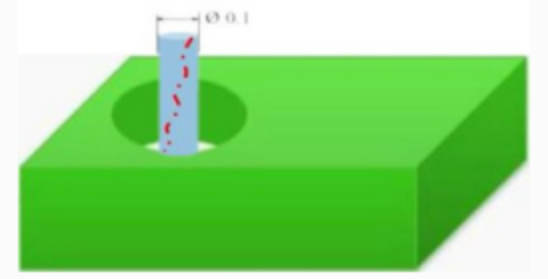Concentricity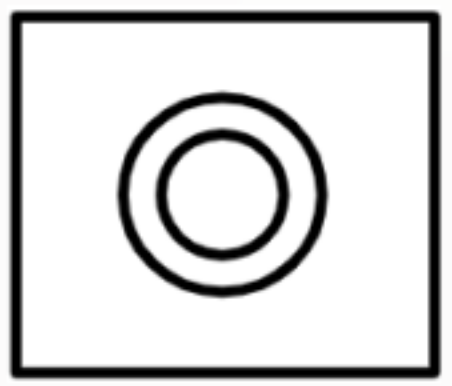Concentricity or coaxiality indicates that the centerline of the tolerated circle lies in a tolerance zone which is a circle with a diameter of the tolerance value. This circle has the same centerline as the datum.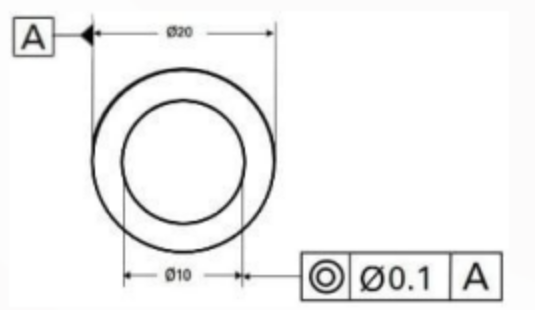Example of a drawing entry:The tolerancezone of this example would look like this: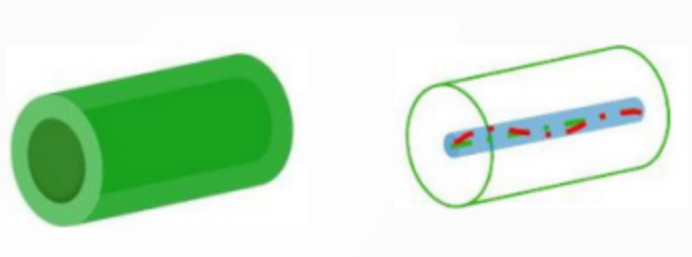Run-out tolerances

 Radial runout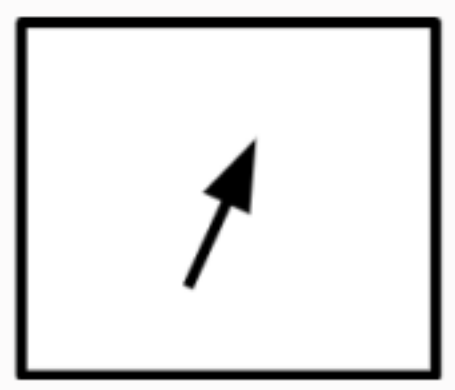The part should be rotated around datum A, a measurement gauge is pressed to the surface of the tolerated cylinder and the gauge reading is set to zero. By rotating the part, the gauge indicates if there is a difference in height between the different points on the rotated circumference. This difference in height is the value of the run out. Example of a drawing entry: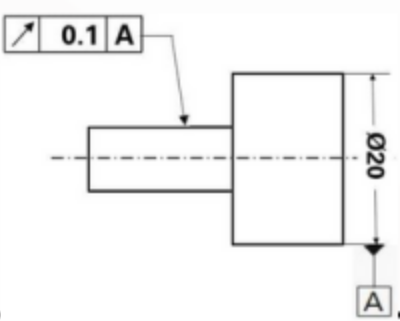The tolerancezone of this example would look like this: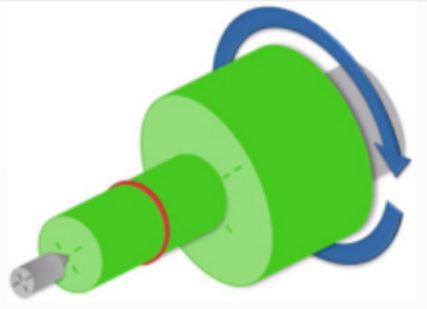Total radial runout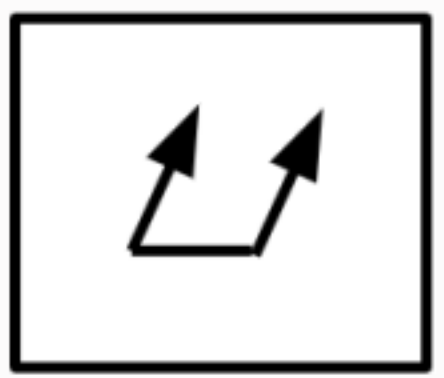The measurement method of the total runout is similar to this of the circular run out, but here, the evaluation is done on the complete cylinder and not on only one section of it. Example of a drawing entry: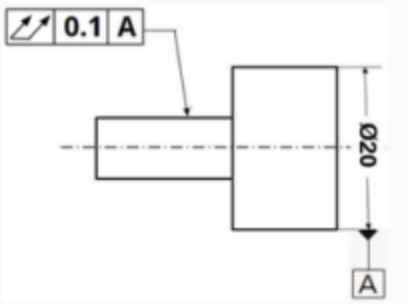The tolerancezone of this example would look like this: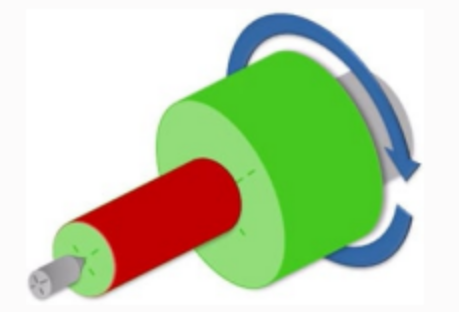Axial runouthere, the tolerated feature is not a cylinder, but a planar surface.The evaluation is carried out on each circle on the surface independently.Example of a drawing entry: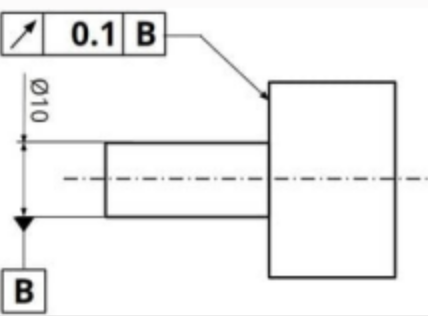The tolerancezone of this example would look like this: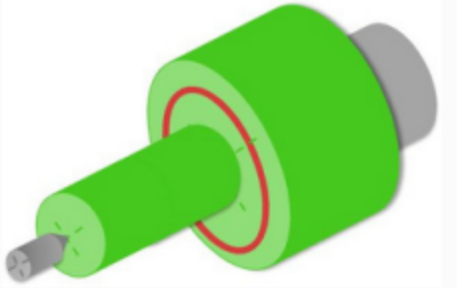Total axial runouthere, the tolerated feature is not a cylinder, but a planar surface. The evaluation is carried out on the complete tolerated feature. Example of a drawing entry: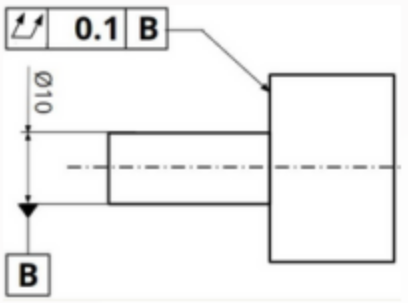The tolerancezone of this example would look like this: